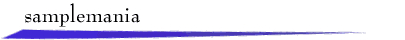## Fine Print

A band-limited signal x(t) that contains no frequencies above wm can in theory be perfectly reconstructed from a sampled signal xs(t) that is sampled at a frequency ws > 2wm. However, the sampling and reconstruction process is complicated, and there are difficulties inherent in the representation and display of continuous-time signals on a computer.

### Pixels Aren't Points

To allow the user to modify signal values with the mouse, SampleMania represents continuous-time signals internally as discrete-time signals with one sample per pixel. Consequently, a smooth continuous-time signal such as a sinusoid behaves, at very fine granularities, like a digital signal with very small jumps, as shown below.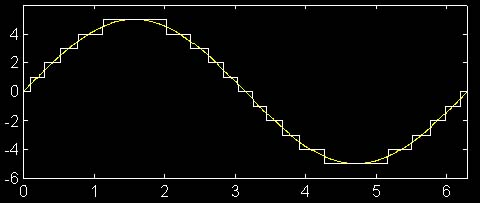The result of this approximation is additional high-frequency signal content that would not be present in the real continuous-time signal, as illustrated by the magnitude spectra below. The spectrum on the left represents the pure sinusoid; the spectrum on the right represents the quantized version.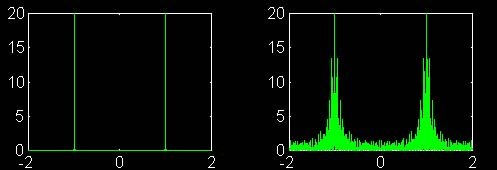### SampleMania Signals Are Finite in Extent

Another approximation is that the "Sinc" and "Sine" buttons generate only windowed versions of the sinc and sine signals. That is, even though the sine and sinc functions are infinite in extent, their representations in SampleMania are zero outside the display area. A property of the Fourier transform is that finite-extent signals have infinite-extent transforms. Similarly, because of duality, signals with finite-extent transforms must be infinite in extent. Consider the case of a rectangular pulse signal and its transform, the sinc: as the pulse becomes taller and narrower, the sinc grows wider, until the limiting case where the pulse becomes an infinite-height impulse and the sinc becomes an infinite-extent constant signal. Conversely, as the pulse becomes wider and approaches a constant signal, the sinc grows taller and narrower, tending toward an impulse. The illustration below shows two rectangular pulse signals (at left) and their corresponding magnitude spectra (at right).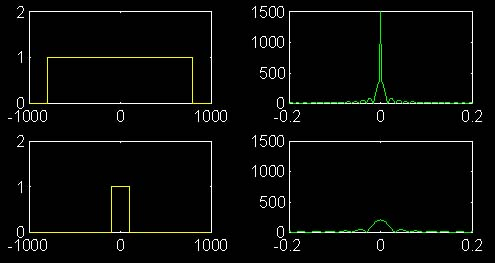As a result, the "sinc" signal displayed by SampleMania is actually a sinc that has been multiplied by a rectangular window in the time domain, zeroing out the small ripples that extend to infinity in the original signal. Because of the modulation property, this multiplication in time is equivalent to convolution with another sinc function in the frequency domain. This tends to distort and "smear" the magnitude spectrum. Because the sinc function never drops off to zero, the magnitude spectrum of our windowed function is never identically zero, resulting in non-zero "ripples" of distortion that extend to infinity. (Note that if the width of our rectangular window approaches infinity, the sinc with which the desired magnitude spectrum is convolved approaches an impulse, and the amount of distortion decreases.) Although the effect of these ripples is small, when the signal is sampled they are enhanced via aliasing, resulting in a significant non-zero offset in the magnitude spectrum of the sampled sinc function. The plots below illustrate the effect of windowing on the magnitude spectrum (in green) of the sinc function. The first sinc signal extends to t = +/- 1000; the second sinc extends only to +/- 300. Note the additional high-frequency ripples and poorer approximation of a rectangular pulse in the spectrum of the second signal.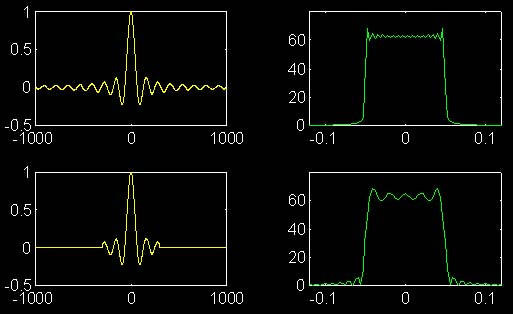### All Magnitude Spectra Are Not Created Equal

It is sometimes noticeable that the "windowed" region of the magnitude spectrum of the sampled signal (impulse train) and the spectrum of the reconstructed signal don't quite match. There are several reasons for this. First, the reconstruction in SampleMania is computed not in the frequency domain with a low-pass filter, but in the time domain via convolution with a sinc (this is done in order to avoid the additional time that would be required to compute an inverse Fourier transform). Although these operations are equivalent in theory, imprecision in the computation of the sinc, as well as the fact that the "sinc" signal used is actually zero outside of the display window, can result in an imperfect reconstruction.

More significantly, the magnitude spectrum of the sampled signal is computed using a slightly different algorithm than the spectra of the signals x(t) and xr(t). The reason is that the sampling frequency can optionally be specified as an integer number of radians per second, meaning that the number of screen pixels in each sampling period is irrational. As a result, if a standard display procedure were used, for the periodic magnitude spectrum of the impulse train, the spectrum values used to compute corresponding pixel positions in successive periods of the signal would be slightly different. The result would be a graph that doesn't look periodic. In the example below, a sine function with period 2p is shown. Superimposed on the graph of the function are "pixels" spaced every unit along the x-axis. When the graph of the continuous function is removed, the resulting pixels do not appear to represent a periodic signal.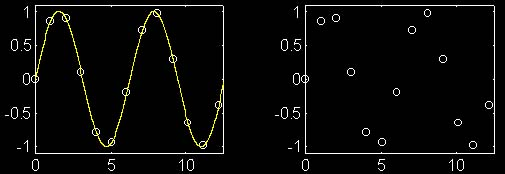To compensate for this and emphasize the basic concepts that SampleMania is trying to convey, a particular method is used for rounding to integer pixel positions when displaying the magnitude spectrum of the sampled signal. This algorithm can result in small discrepancies between the spectra of the sampled and reconstructed signals.